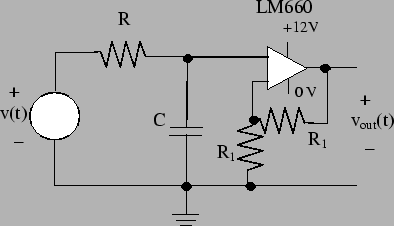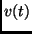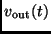Next: RC Circuit Up: Output Compare Interrupts Previous: What is Pulse Width

## How to use a PWM signal to generate an analog voltage

Figure 34 shows a capacitive circuit. This circuit will generate an analog voltage when the applied voltage is a PWM signal.The circuit in figure 34 is driven by a PWM signal,. The remainder of the circuit is divided into two parts. The first part is the RC circuit combination. This part transforms the PWM input signal into an analog voltage. The second part is the op-amp circuit that generates the output voltage. This op-amp circuit is used to buffer the RC circuit from any load that has been put across the circuit's output. We will now discuss how the RC and op-amp parts of this circuit work.

Bill Goodwine 2002-09-29